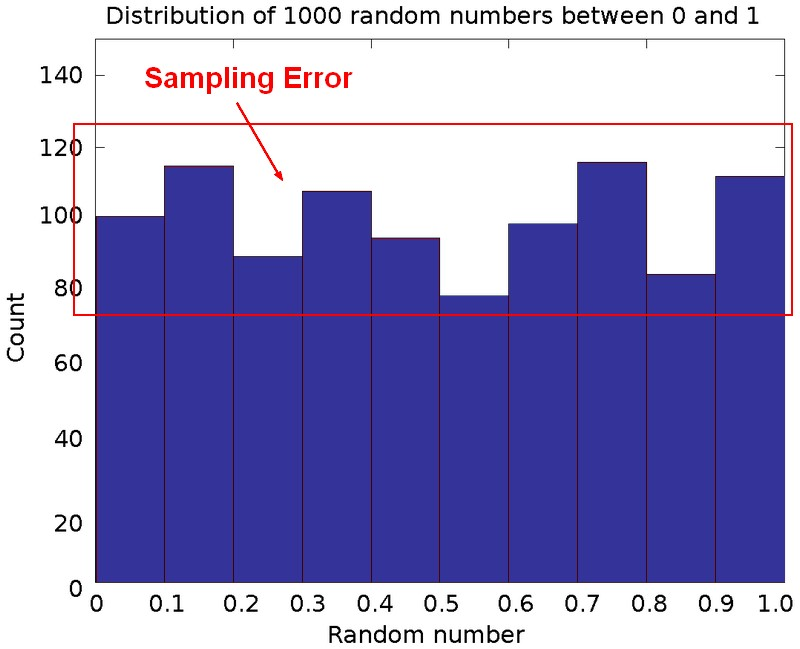# Statistics - Sampling Error

The sampling error is the inaccuracy that results from estimating using a sample, rather than the entire population.

The Sampling error is the difference between the population and the sample.

Whenever a sample is used instead of the entire population, the results are merely estimates and therefore have some chance of being incorrect. This is called sampling error.

Sampling error implies that statistics will vary from one study to the next.

Sampling error is due to chance.

## Example

• Even the random histogram is not perfectly random, there is some fluctuation due to sampling error.
• From several samples of the same population, you will get a little bit of fluctuation that's just due to chance. That's sampling error
• a mean of 12.03
• a mean of 12.9
• a mean of 14.13

## Calculation

Sampling error depends:

• on the size of the sample, relative to the size of the population. As sample size increases, sampling error decreases
• on the variance in the population. As variance increases, sampling error increases

Sampling error is estimated through the Standard error calculation.

## Sample Size

Sampling error, and therefore standard error, is largely determined by sample size.

If I have small samples relative to large populations, I'm going to have a large degree of sampling error.

The normal distribution when sample size is only 10 (first distribution in the upper left corner), is much much wider than when we have a really large sample (such as 1000 for the last distribution in the lower down corner).

That's because standard error is the standard deviation of this sampling distribution.

As the sample get larger, they're going to squeeze around the mean and the standard error is going to get very low.

This points to the problem of NSHT being bias by sample size.

Sample size drives and determine sampling error, influence the t-value and the p-value.

Recommended PagesStatistics

is a scientific discipline devoted to the study of data. is the art of extracting information from data. From Data to Information to Knowledge. No learning. lies lies, damned lies, and statistics....Statistics - (Data Set|Sample)

Because of the difficulties of obtaining information all units in a population, it is common to use a small,random and representative subset of the population called a sample. A sample is a smaller,...Statistics - (Estimation|Approximation)

In statistics, it's always impossible to do experimentation on the entire population. Sample of this population are then used to estimate characteristic of this population (ie the statistics). A sample...Statistics - (dependent|paired sample) t-test

A dependent t-test is appropriate when: we have the same people measured twice. the same subject are been compared (ex: Pre/Post Design) or two samples are matched at the level of individual subjects...Statistics - Chance

The chance effect in Statistics is represented by the sampling error. When something is unsystematic, it's then due to chance. If an error is systematic, it's a bias. The chance probability is: 50%...Statistics - Confidence Interval

The definition of a confidence interval says that under repeated experiments 95% of the time this confidence interval will contain the true statistic (mean, ...). A 95% confidence interval is defined...Statistics - NHST Errors

Probability of Type I errors increases when researchers conduct multiple NHSTs. A Type I error means that the null hypothesis is true, but you reject the null hypothesis. If you set your p-level for...Statistics - Sample size (N)

The size of the sample. Ie the count of case. Sample size drives and determine sampling error, influence the t-value and the p-value.Statistics - Standard Error (SE)

Standard Error is a measure of precision for a statistic (slope, intercept or custom calculations). Standard error is an estimate of amount of sampling error as we typically don’t know the population...Statistics - Statistic

In general, each statistic is an estimate of a parameter, whose value is not known exactly. Every number found using a sample is just an (approximation|estimation) of a parameter (the truth). in descriptive...